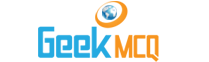Engineering :: Heat Engines

1.  Three states of matter are distinguished with repect to molecules by
 A. The number B. The atoms in molecules C. The orientation of molecules D. Characteristics of the motion of molecules E. Energy of molecules

2.  The molecules move in a solid
 A. At random B. In circular motion C. Back and forth like tiny pendulum D. In irregular motion E. None of above

3.  Sensible heat is
 A. That heat which causes change of state B. That heat which produces a rise of temperature C. That heat which produces a rise of temperature D. That can only be sensed by a thermometer E. None of the above

4.  Specific heat is
 A. The amount of heat required to raise the temperature of a unit quantity of substance through 1?C B. The amount of heat requred to change the state or condition under which a substance exists without changing its temperature C. The ratio of quantity of heat required to raise the temperature of a given weight of any substance through 1?C to the quantity of heat required to raise the temperature of same weight of water through 1?C D. The heat sensed by a mercury thermometer E. None of the above

5.  Working in the cryogenic range means dealing with temperatures
 A. Between - 190?C and absolute zero B. Below zero degree centigrade C. Below absolute zero D. Between zero degree centigrade and 190?C E. Prevailing normally in the atomsphere

6.  Latent heat is
 A. Heat cannot be detected B. Heat that does not follow first law of thermodynamics C. Heat required to change the substance from solid to gaseous state D. Heat required to change the state of substance from liquid state to solid state E. Heat required to change the state or condition under which the substance exists

7.  Absolute pressure is
 A. Gauge pressure B. Gauge pressure + 1.03 kg/cm2 C. Gauge pressure-atmospheric pressure D. Gauge pressure + atmospheric pressure E. None of the above

8.  In thermodynamics spontaneous processes are those
 A. Where a difference is observed in any microscopic property of the system between different times or states B. Where a difference is observed in any microscopic property of the system between different times or states C. Which occur in the direction of an intensive property or driving force contained within the system D. Which require an agency outside the system to force the process E. None of the above

9.  Zeroth law of thermodynamics states that
 A. Two systems are always in equilibrium B. If two systems are in equilibrium the third system will also be in equilibrium C. If two systems are in thermal equilibrium with a third system, then they are in equilibrium with each other D. Two systems not in equilibrium with a third system are also not in equilibrium with each other E. None of the above

10.  The Clapeyron equation is applicable for
 A. System in equilibrium B. Change of state C. Change of state when two phases are in equilibrium D. Change of state when water and water vapours are involved E. None of the above